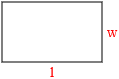# Rectangle Area Calculator

In Euclidean plane geometry, a rectangle is a quadrilateral with four right angles. It can also be defined as an equiangular quadrilateral, since equiangular means that all of its angles are equal.Width (w): Length (l):